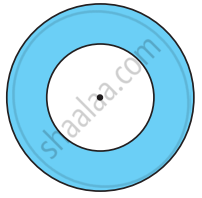# The following figure shows two circles with the same center. The radius of the larger circle is 10 cm and the radius of the smaller circle is 4 cm. Fin:(a) the area of the larger circle - Mathematics

Sum

The following figure shows two circles with the same center. The radius of the larger circle is 10 cm and the radius of the smaller circle is 4 cm.Find:
(a) the area of the larger circle
(b) the area of the smaller circle
(c) the shaded area between the two circles. (π = 3.14)

#### Solution

(a) Radius of the larger circle = 10 cm

So, area of the larger circle= πr2 = 3.14 × 10 × 10 = 314 cm2

(b) Radius of the smaller circle = 4 cm

Area of the smaller circle = πr2 = 3.14 × 4 × 4 = 50.24 cm2

(c) Area of the shaded region = (314 – 50.24) cm2 = 263.76 cm2

Concept: Area of Circle
Is there an error in this question or solution?

Share